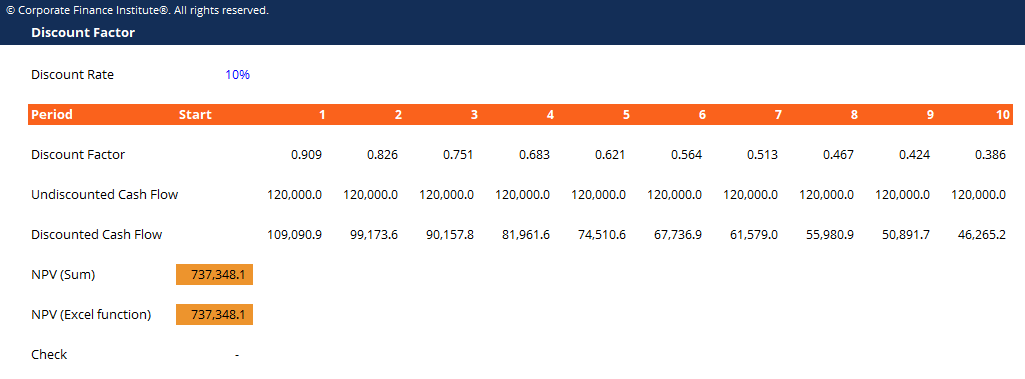# Discount Factor Template

## Discount Factor Template

This discount factor template helps you calculate the amount of discounted cash flows using explicit discount factors.

Here is what the discount factor template looks like:### Discount Factor Template

Discount factor is a decimal number multiplied by a cash flow value to discount it back to the present value.  The discount factor increases over time (meaning the decimal value gets smaller) as the effect of compounding the discount rate builds over time.

Discount factor is an alternative to using the XNPV or XIRR functions in Excel. As opposed to using the XNPV function, manually calculating the discount factor allows you to identify the present value of each individual cash flow.

The discount factor formula is:

Discount Factor = 1 / (1 x (1 + Discount Rate) ^ Period Number)

### More Free Templates

For more resources, check out our business templates library to download numerous free Excel modeling, PowerPoint presentation and Word document templates.

• Excel Modeling Templates
• PowerPoint Presentation Templates
• Transaction Document Templates

### Financial Analyst Certification

Become a certified Financial Modeling and Valuation Analyst (FMVA)® by completing CFI’s online financial modeling classes and training program!